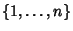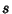## Ryser Formula

A formula for the Permanent of a Matrixwhere the Sum is over all Subsets of, andis the number of elements in. The formula can be optimized by picking the Subsets so that only a single element is changed at a time (which is precisely a Gray Code), reducing the number of additions fromto.

It turns out that the number of disks moved after theth step in the Towers of Hanoi is the same as the element which needs to be added or deleted in theth Addend of the Ryser formula (Gardner 1988, Vardi 1991, p. 111).

References

Gardner, M. The Icosian Game and the Tower of Hanoi.'' Ch. 6 in The Scientific American Book of Mathematical Puzzles & Diversions. New York: Simon and Schuster, 1959.

Knuth, D. E. The Art of Computer Programming, Vol. 2: Seminumerical Algorithms, 3rd ed. Reading, MA: Addison-Wesley, p. 515, 1998.

Nijenhuis, A. and Wilf, H. Chs. 7-8 in Combinatorial Algorithms. New York: Academic Press, 1975.

Vardi, I. Computational Recreations in Mathematica. Reading, MA: Addison-Wesley, p. 111, 1991.